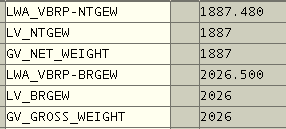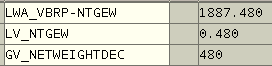SAP ABAP Programming and HANA Database Tutorials Development resources, articles, tutorials, code samples and tools and downloads for ASP.Net, SQL Server, R Script, Windows, Windows Phone, AWS, SAP HANA and ABAP, like SAP UI5, Screen Personas, etc.
 Home Articles News IT Jobs Tools Sample Chapters Trainers Blogs Forums Photos Files

Install SAP Free

# Read Integer and Decimals of a Numeric Value in ABAP Programming

In ABAP programming, to read integer part of a numeric value and to read decimal part of a numeric value is very easy using numeric ABAP function TRUNC and FRAC function.

This ABAP tutorial will demonstrate how to read decimals of a given floating number using ABAP FRAC function. As well as reading decimals, developers will see how to read integer part of numeric values using ABAP TRUNC function. As one last step I'll concatenate integer part and decimals for a specific country using that locale's thousand separator and decimal separator character.

## Read Integers using ABAP TRUNC Function

Here is a sample case where ABAP developers can use TRUNC function to read integer part of numeric values in SAP systems. This ABAP example reads integer part of invoice items net and gross weight information from ABAP VBRP table BRGEW gross weight field and NTGEW net weight field for a given invoice number.

``` DATA :  lv_vbeln TYPE vbeln,  lwa_vbrp TYPE vbrp,  lv_ntgew TYPE i,  lv_brgew TYPE i,  gv_net_weight(20) TYPE c,  gv_gross_weight(20) TYPE c. lv_vbeln = '0030000184'. " Sample invoice number SELECT SINGLE * INTO lwa_vbrp FROM vbrp WHERE vbeln = lv_vbeln. IF sy-subrc EQ 0.  lv_ntgew = TRUNC( lwa_vbrp-ntgew ). " Read integer part  lv_brgew = TRUNC( lwa_vbrp-brgew ). " Read integer part  WRITE lv_ntgew TO gv_net_weight DECIMALS 0 NO-GROUPING. " Write as text  WRITE lv_brgew TO gv_gross_weight DECIMALS 0 NO-GROUPING.  CONDENSE gv_net_weight. " Remove spaces  CONDENSE gv_gross_weight. " Remove spaces  WRITE gv_net_weight. " Write on display  WRITE gv_gross_weight. " Write on display ENDIF. ```This ABAP report will display 1887 as net weight and 2026 as gross weight value ignoring the decimals and only showing integer part of the item's weight data ntgew and brgew field values.

## Read Decimals using ABAP FRAC Function

ABAP programmers sometimes require to read decimals of numeric values for example for custom rounding in ABAP calculations. This ABAP program example will show how to read decimals of invoice item's weight data using ABAP FRAC function.

``` DATA :  lv_vbeln TYPE vbeln,  lwa_vbrp TYPE vbrp,  lv_ntgew TYPE i,  gv_netweightdec(10) TYPE c. lv_vbeln = '0030000184'. " Sample invoice number SELECT SINGLE * INTO lwa_vbrp FROM vbrp WHERE vbeln = lv_vbeln. IF sy-subrc EQ 0.  lv_ntgew = FRAC( lwa_vbrp-ntgew ).  MOVE lv_ntgew TO gv_netweightdec.  CONDENSE gv_netweightdec.  MOVE gv_netweightdec+2(3) TO gv_netweightdec.  WRITE gv_netweightdec. ENDIF. ```Given above ABAP program will display 480 as decimal section of net weight of invoice item. If you use FRAC function for custom rounding, you can compare lv_ntgew with 0.500 for example within your ABAP report. If it is bigger or equal to limit value, you can increase the integer part by one. If it is less than limit value, you can just use the integer part of the numeric value for rounding off.

## Convert Numeric Value using Custom Thousands and Decimal Separator

After you have splitted integer value and decimal value of the floating numeric number in your ABAP report, developers can concatenate these two values using custom thousands and decimals separator. This text representation of numeric values might be a problem for different locales and especially if you are creating Microsoft Excel reports within your ABAP program.

In English thousand separator is "," and decimal separator is "."
For example, in Turkish the case is just the opposite. Thousands separator is "." and the decimal separator is "," in Turkish numeric formatting.

Here is a sample ABAP report to split numeric value into its integer and decimals part and concatenate back using custom separators.

Within the fn_convertTRnumericDisplay ABAP function, you can define your custom thousands and decimal separator as you wish for string representation of the numeric values.

``` DATA :  lv_vbeln TYPE vbeln,  lwa_vbrp TYPE vbrp,  gv_netweight(20) TYPE c. lv_vbeln = '0030000184'. SELECT SINGLE * INTO lwa_vbrp FROM vbrp WHERE vbeln = lv_vbeln. IF sy-subrc EQ 0.  PERFORM fn_convertTRnumericDisplay USING lwa_vbrp-ntgew CHANGING gv_netweight.  WRITE gv_netweight. ENDIF. FORM fn_convertTRnumericDisplay  USING lv_brgew LIKE vbrp-brgew   CHANGING lv_display. DATA :  gv_decimalfrc LIKE vbrp-brgew,  gv_decimaltxt(10) TYPE c,  gv_integerpart TYPE i,  gv_integertxt(13) TYPE n.  gv_integerpart = TRUNC( lv_brgew ).  WRITE gv_integerpart TO gv_integertxt DECIMALS 0. "no-GROUPING.  CONDENSE gv_integertxt.  REPLACE ALL OCCURRENCES OF '.' IN gv_integertxt WITH '.'. " Thousand separator  REPLACE ALL OCCURRENCES OF ',' IN gv_integertxt WITH '.'. " Thousand separator  gv_decimalfrc = FRAC( lv_brgew ).  MOVE gv_decimalfrc TO gv_decimaltxt.  CONDENSE gv_decimaltxt.  MOVE gv_decimaltxt+2(3) TO gv_decimaltxt.  CONCATENATE gv_integertxt ',' gv_decimaltxt INTO lv_display. " Decimal separator ENDFORM. ```

SAP Tutorials

SAP Tutorial

SAP Forums

SAP Tools

SAP Transaction Codes Table

Meetup Sunumu 1